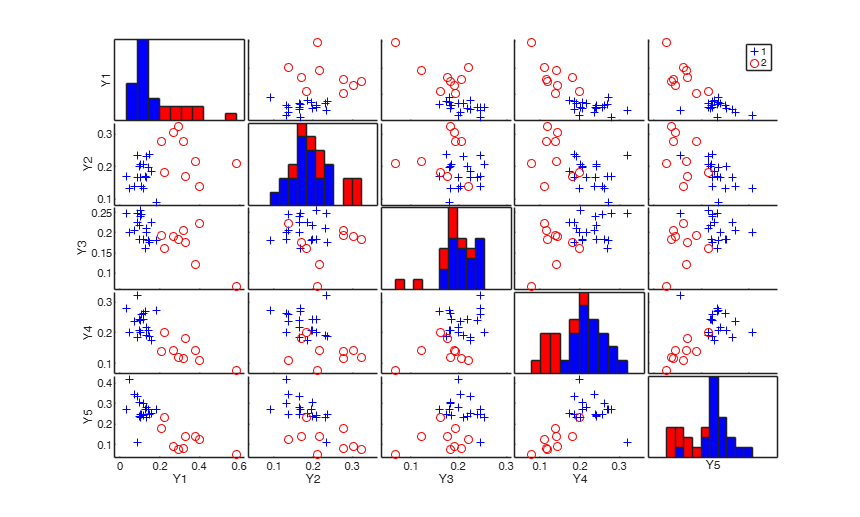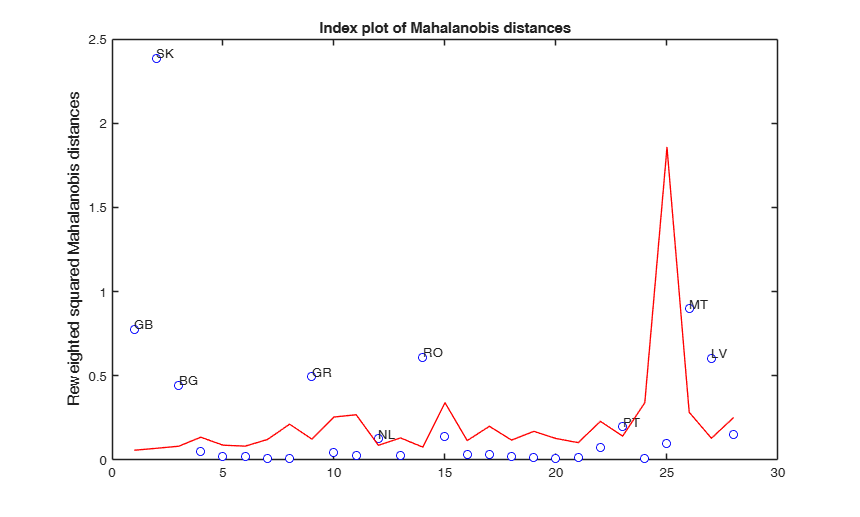# mcdCorAna

mcdCorAna computes Minimum Covariance Determinant in correspondence analysis

## Syntax

• RAW=mcdCorAna(N)example
• RAW=mcdCorAna(N,Name,Value)example
• [RAW,REW]=mcdCorAna(___)example
• [RAW,REW, varargout]=mcdCorAna(___)example

## Description

 RAW =mcdCorAna(N) mcdCorAna with option plots=1.

 RAW =mcdCorAna(N, Name, Value) mcdCorAna with bdp=0.

 [RAW, REW] =mcdCorAna(___) mcdCorAna with option plots.

 [RAW, REW, varargout] =mcdCorAna(___) Raw and reweighted MCD.

## Examples

expand all

### mcdCorAna with option plots=1.

N=[134    76    43    50    49
173    62    20    23    16
67    76    48    36    23
11    21    31    36    52
25    32    57    60    58
32    42    40    67    67
20    35    31    41    41
10    16    23    23    24
54    28    29    30    23
12    19    14    15    20
9    10    14    20    23
52    43    38    47    54
21    36    33    30    36
85    74    55    31    22
3     8    12    12    25
28    33    40    31    45
9    17    23    19    34
18    36    44    35    40
12    24    22    25    37
16    32    35    39    38
28    39    36    41    54
3    15    22    25    24
30    40    28    20    26
8    10    12    13    17
2     1     2     3     3
29    10    16     8     9
47    51    29    19    12
7    19    20    26     9];
rowlab={'GB' 'SK' 'BG' 'IE' 'BE' 'ES' 'PL' 'FI' 'GR' 'HU' 'SI' 'NL' 'IT' 'RO'...
'AT' 'FR' 'HR' 'SE' 'CZ' 'DK' 'DE' 'LT' 'PT' 'EE' 'LU' 'MT' 'LV' 'CY'};
collab={'x1' 'x2' 'x3' 'x4' 'x5'};
Ntable=array2table(N,'RowNames',rowlab,'VariableNames',collab);
RAW=mcdCorAna(Ntable,'plots',1);

###mcdCorAna with bdp=0.

N=[  69    46    41    13    22    18
29    52    45     3     5     3
19    55    47     2     3     1
50    22    19     8    10     7
25    38    33     2     4     3
30     2     1    45     8     2
35     6     5    32     5     2
28    12     7     7     5     4
26    12    11    11     4     3
21     6     4     3     3     2];
rowlab={'Teens' 'PicksYouUp' 'Energy' 'EnjoyLife' ...
'WhenTired' 'Kids' 'Fun' 'Refreshes' ...
'CheersYouUp' 'Relax'};
collab={'Coke' 'V' 'RedBull' 'Fanta' 'Pepsi' 'DietCoke'};
Ntable=array2table(N,'RowNames',rowlab,'VariableNames',collab);
RAW=mcdCorAna(Ntable,'bdp',0);
% Note that in this case RAW.md is equal to
% out.OverviewRows.Inertia./out.OverviewRows.Mass
% out = output from traditional correspondence analysis.
out=CorAna(Ntable,'dispresults',false,'plots',0);
d2=out.OverviewRows.Inertia./out.OverviewRows.Mass;
disp('Square distance of each row profile from the centroid')
disp([RAW.md d2])
The MCD estimates are equal to the classical estimates h=n=1036
Square distance of each row profile from the centroid
0.0962    0.0962
0.2942    0.2942
0.5219    0.5219
0.0932    0.0932
0.2415    0.2415
1.6514    1.6514
0.7965    0.7965
0.1151    0.1151
0.0547    0.0547
0.2517    0.2517



### mcdCorAna with option plots.

N=[134    76    43    50    49
173    62    20    23    16
67    76    48    36    23
11    21    31    36    52
25    32    57    60    58
32    42    40    67    67
20    35    31    41    41
10    16    23    23    24
54    28    29    30    23
12    19    14    15    20
9    10    14    20    23
52    43    38    47    54
21    36    33    30    36
85    74    55    31    22
3     8    12    12    25
28    33    40    31    45
9    17    23    19    34
18    36    44    35    40
12    24    22    25    37
16    32    35    39    38
28    39    36    41    54
3    15    22    25    24
30    40    28    20    26
8    10    12    13    17
2     1     2     3     3
29    10    16     8     9
47    51    29    19    12
7    19    20    26     9];
rowlab={'GB' 'SK' 'BG' 'IE' 'BE' 'ES' 'PL' 'FI' 'GR' 'HU' 'SI' 'NL' 'IT' 'RO'...
'AT' 'FR' 'HR' 'SE' 'CZ' 'DK' 'DE' 'LT' 'PT' 'EE' 'LU' 'MT' 'LV' 'CY'};
collab={'x1' 'x2' 'x3' 'x4' 'x5'};
Ntable=array2table(N,'RowNames',rowlab,'VariableNames',collab);
RAW=mcdCorAna(Ntable,'plots',1);

###Raw and reweighted MCD.

N=[134    76    43    50    49
173    62    20    23    16
67    76    48    36    23
11    21    31    36    52
25    32    57    60    58
32    42    40    67    67
20    35    31    41    41
10    16    23    23    24
54    28    29    30    23
12    19    14    15    20
9    10    14    20    23
52    43    38    47    54
21    36    33    30    36
85    74    55    31    22
3     8    12    12    25
28    33    40    31    45
9    17    23    19    34
18    36    44    35    40
12    24    22    25    37
16    32    35    39    38
28    39    36    41    54
3    15    22    25    24
30    40    28    20    26
8    10    12    13    17
2     1     2     3     3
29    10    16     8     9
47    51    29    19    12
7    19    20    26     9];
rowlab={'GB' 'SK' 'BG' 'IE' 'BE' 'ES' 'PL' 'FI' 'GR' 'HU' 'SI' 'NL' 'IT' 'RO'...
'AT' 'FR' 'HR' 'SE' 'CZ' 'DK' 'DE' 'LT' 'PT' 'EE' 'LU' 'MT' 'LV' 'CY'};
collab={'x1' 'x2' 'x3' 'x4' 'x5'};
Ntable=array2table(N,'RowNames',rowlab,'VariableNames',collab);
[RAW,REW]=mcdCorAna(Ntable,'plots',1);
Total estimated time to complete MCD:  0.28 seconds## Input Arguments

### N — Contingency table (default) or n-by-2 input dataset. 2D Array or Table.

2D array or table which contains the input contingency table (say of size I-by-J) or the original data matrix X.

In this last case N=crosstab(X(:,1),X(:,2)) or N=crosstab(X(:,1),X(:,2)) if X is in table format. As default procedure assumes that the input is a contingency table.

Data Types: table, or array

### Name-Value Pair Arguments

Specify optional comma-separated pairs of Name,Value arguments. Name is the argument name and Value is the corresponding value. Name must appear inside single quotes (' '). You can specify several name and value pair arguments in any order as  Name1,Value1,...,NameN,ValueN.

Example:  'bdp',1/4 , 'nsamp',10000 , 'refsteps',10 , 'reftol',1e-8 , 'refstepsbestr',10 , 'reftolbestr',1e-8 , 'bestr',10 , 'conflev',0.99 , 'plots',1 , 'Lr',{'UK' ... 'IT'} , 'Lc',{'x1' ... 'x5'} , 'msg',false , 'tolMCD',1e-20 , 'findEmpiricalEnvelope',true 

### bdp —Breakdown point.scalar.

(Number between 0 and 0.5) or if it an integer greater than 1 bdp is the number of data points which have to determine the fit The default value is 0.5.

Example:  'bdp',1/4 

Data Types: double

### nsamp —Number of subsamples.scalar.

Number of subsamples of size J which have to be extracted (if not given, default = 1000).

Example:  'nsamp',10000 

Data Types: double

### refsteps —Number of refining iterations.scalar.

Number of refining iterations in each subsample (default = 3).

refsteps = 0 means "raw-subsampling" without iterations.

Example:  'refsteps',10 

Data Types: double

### reftol —Refining steps tolerance.scalar.

Tolerance for the refining steps.

The default value is 1e-6;

Example:  'reftol',1e-8 

Data Types: double

### refstepsbestr —Number of refining iterations.scalar.

Number of refining iterations for each best subset (default = 50).

Example:  'refstepsbestr',10 

Data Types: double

### reftolbestr —Tolerance for refining steps.scalar.

Value of tolerance for the refining steps for each of the best subsets.

The default value is 1e-8;

Example:  'reftolbestr',1e-8 

Data Types: double

### bestr —Number of best solutions to store.scalar.

Number of "best locations" to remember from the subsamples. These will be later iterated until convergence (default=5)

Example:  'bestr',10 

Data Types: double

### conflev —Confidence level.scalar.

Number between 0 and 1 containing confidence level which is used to declare units as outliers.

Usually conflev=0.95, 0.975 0.99 (individual alpha) or 1-0.05/I, 1-0.025/I, 1-0.01/I (simultaneous alpha).

Default value is 0.99 per cent simultaneous

Example:  'conflev',0.99 

Data Types: double

### plots —Plot on the screen.scalar | structure.

If plots is a structure or scalar equal to 1, generates: (1) a plot of Mahalanobis distances against index number. The confidence level used to draw the confidence bands for the MD is given by the input option conflev. If conflev is not specified a nominal 0.975 confidence interval will be used.

(2) a scatter plot matrix with the outliers highlighted.

If plots is a structure it may contain the following fields

Value Description
labeladd

if this option is '1', the outliers in the spm are labelled with their unit row index. The default value is labeladd='', i.e. no label is added.

nameY

cell array of strings containing the labels of the variables. As default value, the labels which are added are Y1, ...Yv.

Example:  'plots',1 

Data Types: double or structure

### Lr —row labels.cell array.

Cell of length I containing the labels of the rows.

Example:  'Lr',{'UK' ... 'IT'} 

Data Types: cell

### Lc —column labels.cell array.

Cell of length J containing the labels of the columns.

Example:  'Lc',{'x1' ... 'x5'} 

Data Types: cell

### msg —Display or not messages on the screen.boolean.

If msg==true (default) messages are displayed on the screen about estimated time to compute the final estimator else no message is displayed on the screen.

Example:  'msg',false 

Data Types: logical

### tolMCD —Tolerance to declare a subset as singular.scalar.

The default value of tolMCD is exp(-50*v).

Example:  'tolMCD',1e-20 

Data Types: double

### findEmpiricalEnvelope —Empirical Confidence level.boolean.

If findEmpiricalEnvelope is true (default is false) the empirical envelope for each Mahalanobis distance of each Profile row of the contingency table is computed, else the empirical envelopes are found just if input option plots=1. In case findEmpiricalEnvelope is false the theoretical envelope is based on the quantiles of the following scaled gamma distribution chi2inv(conflev,(J-1)*(I-1)/I)/n,I,1)./r;

Example:  'findEmpiricalEnvelope',true 

Data Types: Boolean

## Output Arguments

### RAW — description Structure

Structure which contains the following fields

Value Description
h

scalar. The number of observations that have determined the MCD estimator

loc

1 x J vector containing raw MCD location of the data

cov

robust MCD estimate of covariance matrix. It is the raw MCD covariance matrix (multiplied by a finite sample correction factor and an asymptotic consistency factor).

obj

The determinant of the raw MCD covariance matrix.

bsb

k x 1 vector containing the rows of matrix N which contributed to the computation of the MCD estimate of location

md

I x 1 vector containing the estimates of the robust Mahalanobis distances (in squared units). This vector contains the distances of each observation from the raw MCD location of the data, relative to the raw MCD scatter matrix diag(raw MCD location)

outliers

A vector containing the list of the rows declared as outliers using confidence level specified in input scalar conflev.

conflev

Confidence level that was used to declare outliers and to do reweighting.

singsub

Number of subsets without full rank. Notice that out.singsub > 0.1*(number of subsamples) produces a warning

weights

I x 1 vector containing the estimates of the weights.

Weights assume values in the interval [0 1].

Weight is 1 if the associated row fully contributes to compute centroid and covariance matrix. If for a particular row weight is 0.7 it means that the associated row contributes with 70 per cent of its row mass. 0 weight for a particular row it means that the associated row does not participate at all.

Note that sum(N,2)'*RAW.weights=h

N

Original contingency table in array format.

Ntable

Original contingency table in table format.

Y

array I-by-J containing matrix of Profile Rows.

EmpEnv

array of size I-by-1 containing empirical envelopes for each Mahalanobis distance if input option findEmpiricalEnvelope is true or scalar containing quantile which ahse been used to declare the outliers.

class

'mcdCorAna'

### REW — description Structure

Structure which contains the following fields

Value Description
md

I x 1 vector containing the estimates of the robust Mahalanobis distances (in squared units). This vector contains the distances of each observation from the rewighted MCD location of the data, relative to the reweighted MCD scatter matrix diag(reweighted MCD location)

weights

I x 1 vector containing the estimates of the weights.

Weights assume values 0 or 1. Weight is 0 if the associated row has been declared outlier after reweighting.

outliers

A vector containing the list of the rows declared as outliers using confidence level specified in input scalar conflev

Y

array I-by-J containing matrix of Profile Rows.

EmpEnv

array of size I-by-1 containing empirical envelopes for each Mahalanobis distance if input option findEmpiricalEnvelope is true or scalar containing quantile which ahse been used to declare the outliers.

class

'mcdCorAna'